## Thursday, August 04, 2011 ...//### HadCRUT3: 31% of stations saw cooling since 1979

A few days ago, I analyzed the warming trends at all stations included in the HadCRUT3 zipped data that were recently released.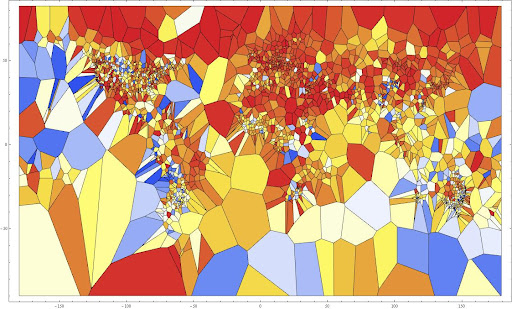Click to zoom in. I chose high contrast colors to make the picture nice. Note that the very red Arctic is actually a very small area on the Earth - which gets artificially expanded in the map whose coordinates are longitude and latitude.

The average station spanned 77 years and showed a warming trend of $0.75\pm 2.35$ °C per century. This wide distribution meant that 30 percent of the stations recorded a cooling. Now I expect that the reader is familiar with the rough methods of my previous article.

So what are the new results? I have simply erased all the data from years prior to 1979 and repeated the same calculations. Almost 4,000 stations contain some data between 1979 - the beginning of the UAH/RSS AMSU satellite era - and 2011. The distribution of the annual-averaged trends per century looks like this: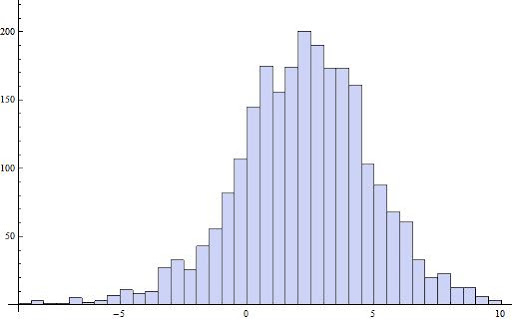The mean value and the standard deviation of the distribution is $2.24\pm 7.04$ °C per century. (Quite generally, an extra comment: my resulting figures for the standard deviations are much larger than the half-widths of the "bell curves" because the distributions are not normal and the large values of the trend actually contribute much more than they do in the normal distribution.)Note that UAH AMSU gives the global warming trend of $1.4$ °C per century since 1979 (you shouldn't extrapolate it - the 1979-2011 interval is a cherry-picked period with some above-average warming, so the actual temperature change per century is always smaller than what the trend calculated from a shorter period indicates!), so the weather stations are exaggerating the warming trend by about 50%. Most likely, it's due to systematic biases of the surface weather stations.

However, you should notice that the standard deviation is $7.04$ °C per century. This local variability is obviously natural; only the mean value may be shifted due to various effects that may include a nonzero contribution of the human activity (but there are surely other contributions as well - and they may dominate). As a result of this local variability, it is completely normal to find stations that indicate a warming trend or cooling trend comparable to $10$ °C per century from 1979. Again, you may want to express this quantity as $1$ °C per decade if you're at risk that the trend will lead you to incorrectly extrapolate.

And the percentage of the cooling station/month combinations since 1979 is 4596/14597 which is 31.5 percent, even higher than the percentage we calculate when we didn't truncate anything before 1979. On one hand, we could have expected the percentage to be closer to 50 percent because shorter periods of time contain a higher fraction of the "noise". On the other hand, we could have expected the fraction to be closer to 0 percent because some other data may lead some people to believe that "the warming since 1979 is big and important and global".

So these two effects approximately cancel. The percentage of the cooling stations has been over 30 percent even since 1979.

An operation I did in the previous article and that I will repeat is the separation into months. If you only compute the warming or cooling trends over all Januaries or all Februaries and so on, and you count the percentages of the month/station combinations with a given warming trend per century, the twelve histograms will look like this: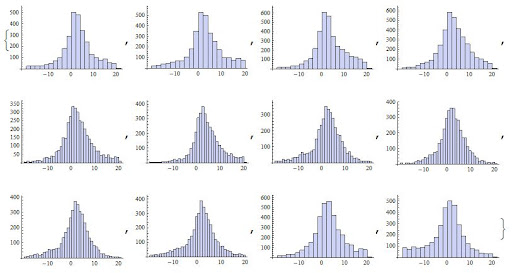You see that these bell curves are not that smooth and nice as we used to have them in the untruncated analysis: after all, the amount of data is limited so the "noise" upon the optimized curves has increased. The twelve histograms may surely look unreadable to you. So here you can see the table for the 12 months. The columns indicate the number of stations that contained relevant data for the station; the mean value of the warming trend; and the standard deviation of the warming trend.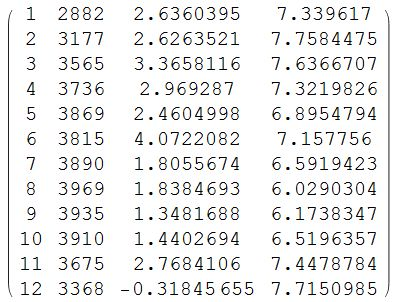The standard deviations are kind of constant - going from the maximum of $7.75$ °C per century in February - the same month as in the untruncated data - down to $6.03$ °C per century in August (it used to be September). The local variability of the trends is very large, indeed.

But the mean values of the trends are violent and completely different than what we used to have. Recall that in the untruncated data, February saw the fastest mean warming trend of $1.1$ °C per century and September saw the slowest one, around 50 percent of this value. However, this is what you get with the truncation to 1979-2011: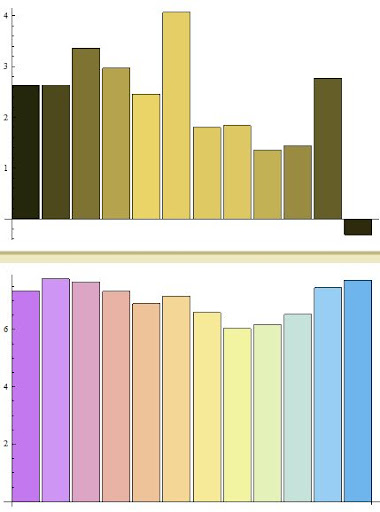The fastest warming trend - when it comes to the mean value - is June (thanks, Howard) which saw $4.07$ °C of warming per century in this interval. On the other hand, the slowest warming was actually cooling: the trend recorded in Winter was actually negative, $-0.32$ °C per century.

So the June warming trend has been huge; the December trend was cooling. In other words, during the last 32 years, a majority of the stations has seen increasingly warm weather in June but the temperature in December didn't change. Did you know? Would you know about this conclusion if I didn't tell you what the mathematical analysis of the data says?

I doubt it. It's because 32 years is short enough a period of time so that all these "trends" are still dominated by noise. There is almost certainly no "universal" explanation why December should see a much lower warming trend than other months. It just happened to be so. Those trends calculated for individual weather stations still show mostly the weather in the last 32 years, not the climate.

For the same reason, you should be very cautious about the interpretation of their averages as well.

Truncating to 1995-2011

I have also repeated the same calculation truncated to 1995-2011, i.e. 16+ years. The mean values of the monthly warming trends are pretty much 12 random numbers between $0$ °C per century for February - yes, February is the slowest warming in this case, showing that there's no "universal pattern", at least not at sub-century timescales - to $4$ °C per century for Aprils (not far!). The average is about $2$ °C per century.

The standard deviations are about $8$ °C, pretty much independent of the month, so the average standard deviation is the same thing.

About 39% of the 21,000+ month/station combinations that reported a trend yielded a negative warming trend: if you only look at 16 years, the number of places that see a warming trend is already nearly balanced with the number of places that see a cooling trend. The histograms are similar but wider (and having an even smaller mean value / standard deviation ratio), the trends for 12 months are chaotic and described above.

But the Voronoi map is something I won't omit: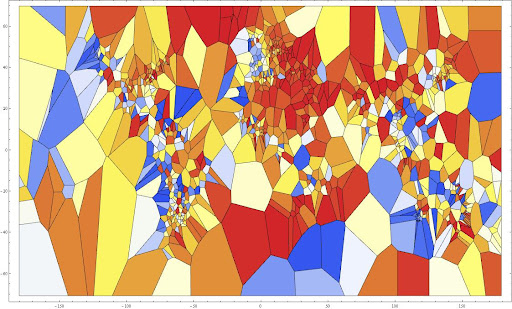Warming trends for stations that reported some data between 1995 and 2011.

Since 2001

With the 2001-2011 filter, the histograms begin to resemble a pyramid rather than a bell curve - interesting. The month-restricted trends are from $0$ to $4$ but the average plus minus error is just $0.8\pm 12.7$ °C per century - the noise is huge. Note that the satellites indicate a negative mean value of this trend - a cooling.

47% of the 17,000 relevant station/month combinations report a cooling. The map looks much more blue - colder - now: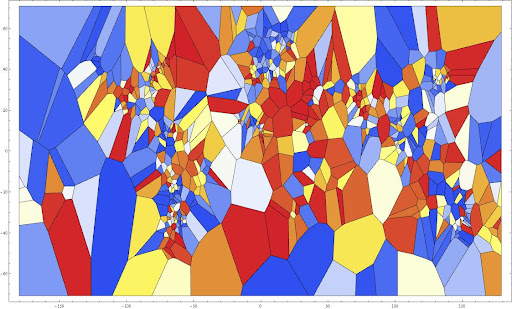Warming or cooling trends across the globe in the 2001-2011 era.

I haven't mentioned one thing that may be confusing. The colorful maps are not periodic in the horizontal direction or (i.e.) continuous on the left-right boundary - on the 180° longitude. That's because my calculational method doesn't identify these two lines in any way and the raw data are not "continuous enough" to know about the identification of these two line segments on the picture, either.

Again: why the trends come out huge for short periods of time

Many people, including skeptics, react totally irrationally when they hear the relatively large values of the trends that were calculated from short enough a period of time. But it is totally inevitable, by basic laws of mathematics and physics, that the trends calculated from noisy data will inevitably end up with huge values if the periods of time are short.

I have explained this point many times but again. Think of the temperature in a region as a random walk or Brownian motion. Brownian motion is a chaotic motion of a small dust particle in water. It's chaotic because the water molecules are hitting it at random moments and from random directions.

Most of these collisions cancel out. But not all of them cancel out. If you have $N$ collisions, approximately $\sqrt{N}$ of them will not cancel out. That's a basic result about the width of the binomial distribution. So after time $t\sim N$, the dust particle will move roughly by $\sqrt{N}\sim\sqrt{t}$ in a random direction.

The overall change of the location - and, analogously, the overall change of the temperature - inevitably increases if you increase the length of the time interval.

What happens with the "average velocity" of the dust particle - or, analogously, the warming trend? Well, you must divide the overall change of the location by $\Delta t$. You will get

$|\langle \vec v \rangle| \sim \frac{\sqrt{\Delta t}}{\Delta t} \sim \frac{1}{\sqrt{\Delta t}}$
or, analogously,
$\left|\left\langle \frac{\Delta\,\,{\rm temperature} }{\Delta t} \right\rangle\right| \sim \frac{\sqrt{\Delta t}}{\Delta t} \sim \frac{1}{\sqrt{\Delta t}}$
where $t$ is time. In the real world, the 0.5-th power (the square root) isn't necessarily accurate. But the best approximation does include some exponent strictly between 0 and 1. So the lesson is that the overall temperature (or location) change does increase with time $t$; however, the average velocity or the average temperature trend calculated from the data inevitably decreases with time $t$.

So all the high figures for the trends that you see above are completely natural. This is how Nature and mathematics work. If you calculated the temperature trends at different places of the globe during a 32-year period during Jurassic, you would get pretty much the same result. The standard deviation of the trend would be about $7$ °C per century and the global trend would be comparable to 1/3 of this standard deviation.

A spin foam. The physical theory of a spin foam is completely wrong and worthless but the research has led to the creation of the video above - the only tangible result of the spin foam research. It's this video that some people find analogous to the Voronoi diagrams.

Those are just natural variations that Nature contains and everyone who wants to understand the climate and the weather should first learn something about the natural variability that the changing weather brings to various places of the globe - as well as their average. Despite the widespread popular misconceptions - that the industry of alarm systematically abuses - it is not true that all this noise averages out in Nature.

This perfect cancellation just doesn't work. In the same way, if you throw dice 5,999 times and you only get 940 times "6", instead of 1000 times as you expected, it does not mean that when you throw it for the 6000th time, you have to get a "6". ;-) You still get "6" with the probability of 1/6 only. Nature exhibits absolutely no respect for egalitarianism, affirmative action, quota, political correctness, or leftwingers, for that matter. And neither do I.

Wrong terminology in all figures for the standard deviation

Bill Zajc has discovered an error that affects all values of the standard deviation indicated in both articles. What I called "standard deviation" was actually the "root mean square", $RMS$. If you want to calculate the actual value of $SD$, it is given by
$SD^2 = RMS^2 - \langle TREND \rangle^2$ In the worst cases, those with the highest $\langle TREND \rangle / RMS$, this corresponds to a nearly 10% error: for example, $2.35$ drops to $2.2$ °C / century or so. My sloppy calculation of the "standard deviation" was of course assuming that the distributions had a vanishing mean value, so it was a calculation of $RMS$.

The error of my "standard deviation" for the "very speedy warming" months is sometimes even somewhat larger than 10%. I don't have the energy to redo all these calculations - it's very time-consuming and CPU-time-consuming. Thanks to Bill.